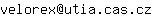Ústav teorie informace a automatizace

## Probabilistic Compositional Models: solution of an equivalence problem

Václav Kratochvíl
Typ obhajoby:
Ph.D.
Datum konání:
24.02.2012
Místo:
místnost č 112, Trojanova 13, Praha 2
Mail:Stav:
obhájeno
An integral part of the work with multidimensional probabilistic models, and in particular their learning, is the perfect knowledge of the conditional independence relations of the model. The knowledge of conditional independence relations is understood here as a list of conditional independence relations valid for the model and induced by its structure. However, the representation these relations by such a structure is imperfect - two or more structures may represent the same independence relations - they are equivalent. The equivalence problem can be summed up in the following three questions: (i) how can we recognize whether two structures represent the same set of independence relations; (ii) how can we transform any one structure into another equivalent one in terms of some elementary operations; and (iii) can we generate all structures equivalent with a given one? This thesis presents the complete solution to equivalence problem. We illustrated its usage and, above that, we found a unique representative of a class of equivalent structures.
03.05.2018 - 08:01